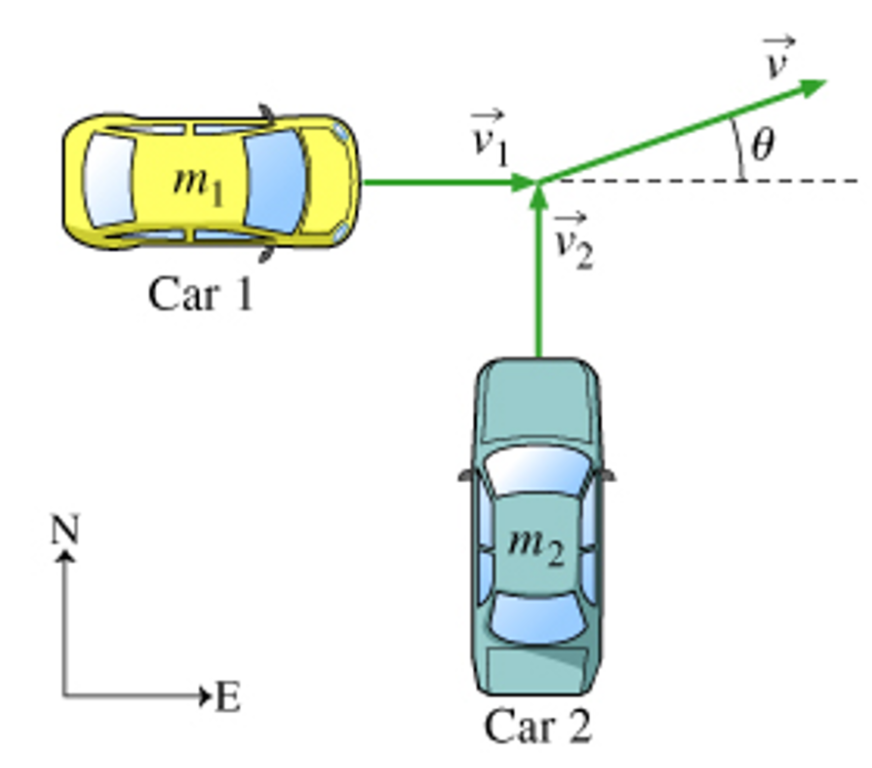# Problem: In this problem we will consider the collision of two cars initially moving at right angles. We assume that after the collision the cars stick together and travel off as a single unit. The collision is therefore completely inelastic. Two cars of masses m1 and m2 collide at an intersection. Before the collision, car 1 was traveling eastward at a speed of v1, and car 2 was traveling northward at a speed of v2. (Figure 1) After the collision, the two cars stick together and travel off in the direction shown.(a) Write the momentum conservation equation for the east-west components. Express your answer in terms of v1, m1, m2, θ, and v, the speed of the 2-car unit after the collision.(b) Write the momentum conservation equation for the north-south components. Express your answer in terms of v1, m1, m2, θ, and v, the speed of the 2-car unit after the collision.(c) Find the magnitude of v , that is, the speed v of the two-car unit after the collision. Express your answer in terms of v1, m1, m2, and v2.(d) Find the tangent of the angle. Express your answer in terms of v1, m1, m2, and v2.

###### FREE Expert Solution

The momentum of a moving object is given by:

$\overline{){\mathbf{p}}{\mathbf{=}}{\mathbf{m}}{\mathbf{v}}}$

Let the y-axis point to the north and the y-axis point to the left.

(a)

Momentum before collision = momentum after collision

m1v1 + m2(0) = (m1 + m2) v cos θ###### Problem Details

In this problem we will consider the collision of two cars initially moving at right angles. We assume that after the collision the cars stick together and travel off as a single unit. The collision is therefore completely inelastic. Two cars of masses m1 and m2 collide at an intersection. Before the collision, car 1 was traveling eastward at a speed of v1, and car 2 was traveling northward at a speed of v2. (Figure 1) After the collision, the two cars stick together and travel off in the direction shown.(a) Write the momentum conservation equation for the east-west components. Express your answer in terms of v1, m1, m2, θ, and v, the speed of the 2-car unit after the collision.

(b) Write the momentum conservation equation for the north-south components. Express your answer in terms of v1, m1, m2, θ, and v, the speed of the 2-car unit after the collision.

(c) Find the magnitude of v , that is, the speed v of the two-car unit after the collision. Express your answer in terms of v1, m1, m2, and v2.

(d) Find the tangent of the angle. Express your answer in terms of v1, m1, m2, and v2.